# Convert Single Precision Lookup Table to Half Precision

This example shows how to convert a single-precision lookup table to use half precision. Half precision is the storage type; the lookup table computations continue to be performed using single precision. After the conversion, the examples halves the memory size of the Lookup Table blocks while keeping the desired system performance.

### Task 1: Simulate and Obtain Baseline

1. Open the `airflow_calc` subsystem of the `sldemo_fuelsys` example model. It contains the Lookup Table blocks with the single precision table and breakpoint data to be converted to half precision.

```load_system('sldemo_fuelsys'); open_system('sldemo_fuelsys/fuel_rate_control/airflow_calc'); ```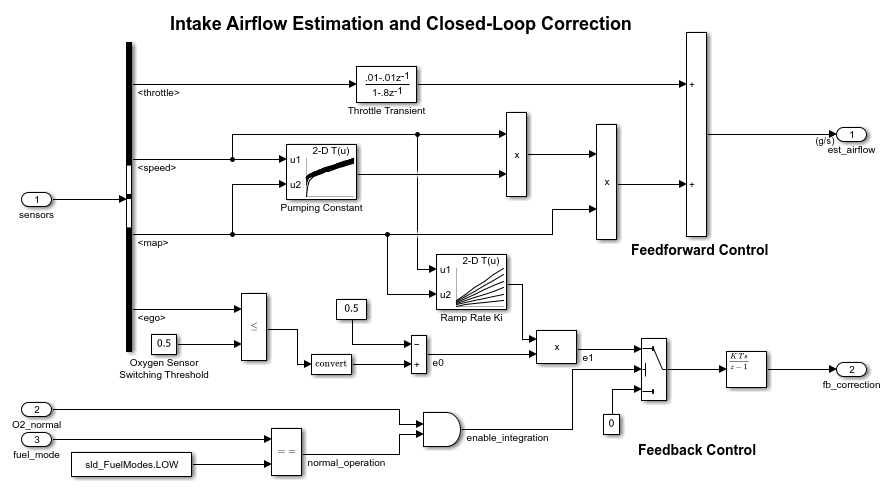2. In MATLAB®, change your current folder to a writable folder.

3. Check that the table and breakpoints Pumping Constant and Ramp Rate Ki Lookup Table block data types are set as single.

4. Select output signals for both Lookup Table blocks. Mark them for logging. Simulate the model in normal mode and use the outputs of this run as a baseline.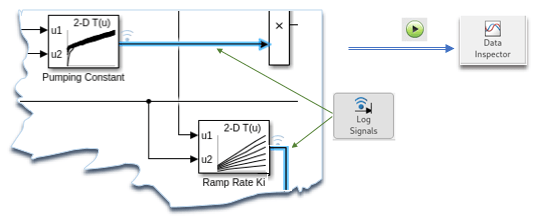### Task 2: Analyze and Convert Data to Half

1. Get the table and breakpoint variable data from the model workspace for the specified Lookup Table block. For example, using the 'Pumping Constant' block:

```mdlWks = get_param('sldemo_fuelsys','ModelWorkspace'); currentBlk = 'sldemo_fuelsys/fuel_rate_control/airflow_calc/Pumping Constant'; ```

2. Analyze the table data and convert it to half precision if it can fit in the range of half precision type [-65504, 65504]. In Simulation Data Inspector, simulate the model and compare the logged output of the current block with the baseline. Verify that the output is within the specified absolute tolerance and relative tolerance. For example, this code sets both tolerances to | `0.01` |.

```expath = fullfile(matlabroot,'examples','fixedpoint','main'); addpath(expath); % Add the folder containing the supporting file to the path numBytesSaved(1) = analyzeDataConvertToHalf(mdlWks, currentBlk, 'Table'); ```

3. Repeat step 2 of Task 2 for data of each of the breakpoints of the current block. The breakpoints data should remain monotonically increasing after converting from single to half precision.

```numBytesSaved(2) = analyzeDataConvertToHalf(mdlWks, currentBlk, 'BreakpointsForDimension1'); numBytesSaved(3) = analyzeDataConvertToHalf(mdlWks, currentBlk, 'BreakpointsForDimension2'); totalBytesSaved = sum(numBytesSaved(1:3)); ```

4. Repeat steps 2 and 3 of Task 2 for the remaining Lookup Table blocks in the same subsystem. Then, examine the number of bytes saved for the table and breakpoints of the n-D Lookup Table blocks.

```currentBlk = 'sldemo_fuelsys/fuel_rate_control/airflow_calc/Ramp Rate Ki'; numBytesSaved(4) = analyzeDataConvertToHalf(mdlWks, currentBlk, 'Table'); numBytesSaved(5) = analyzeDataConvertToHalf(mdlWks, currentBlk, 'BreakpointsForDimension1'); numBytesSaved(6) = analyzeDataConvertToHalf(mdlWks, currentBlk, 'BreakpointsForDimension2'), ```
```numBytesSaved = 684 36 38 72 12 12 ```
```totalBytesSaved = totalBytesSaved + sum(numBytesSaved(4:6)), ```
```totalBytesSaved = 854 ```

### Task 3: Simulate and Compare

1. Simulate the converted model with half precision table and breakpoints.

2. Compare the 'fuel', 'air_fuel_ratio', etc. signals with the baseline in the Scope block and in the Simulation Data Inspector. Observe that the desired performance is still achieved.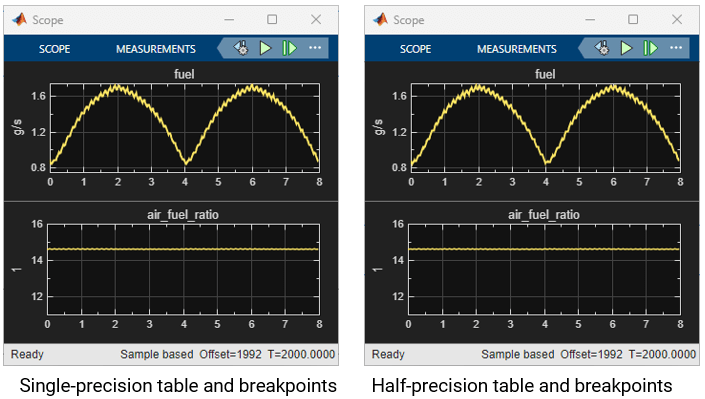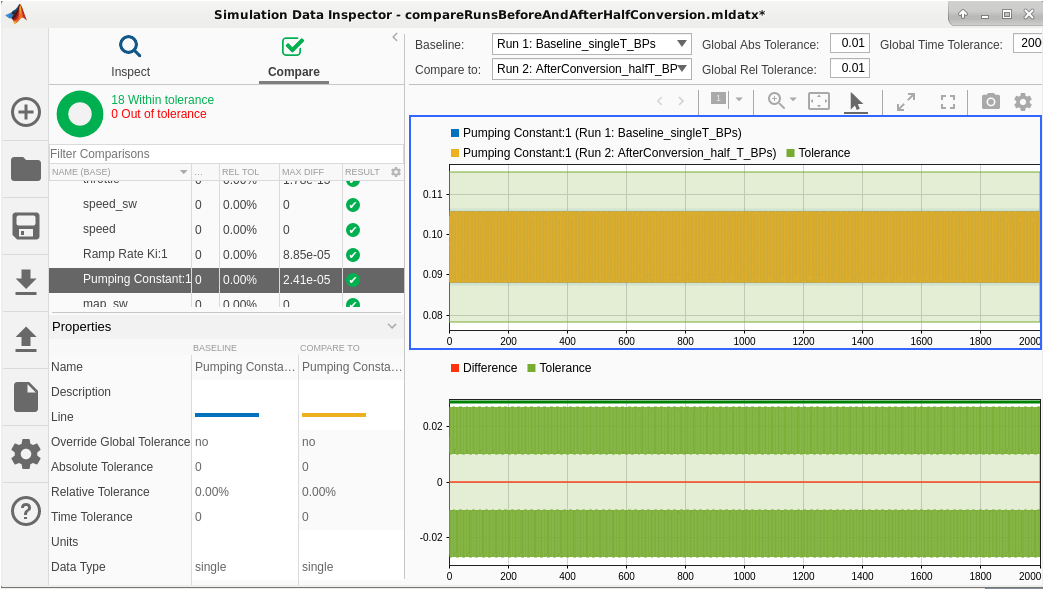3. Save the model with a different name in the writable folder.

### Task 4: Generate Code and Verify the Memory Optimization

1. Right-click the fuel_rate_control subsystem and select C/C++ Code > Build This Subsystem. To generate code, click Build in the Build code for Subsystem dialog box.

2. When the build finishes processing, a code generation report displays.

3. Click the `half_type.h` and `fuel_rate_control.c` files. Notice the half precision (`real16_T`) type definition, the `real16_T` type table, and breakpoint pointers in the `look2_ifbhlfthDfIf_linlca` function call interface.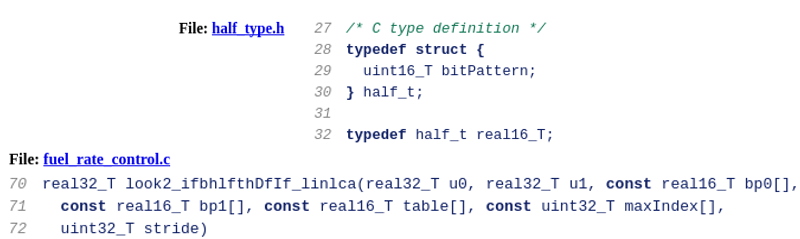4. Open the `fuel_rate_control.h` file and observe that 854 bytes have been saved by using half precision as the storage type for table and breakpoints.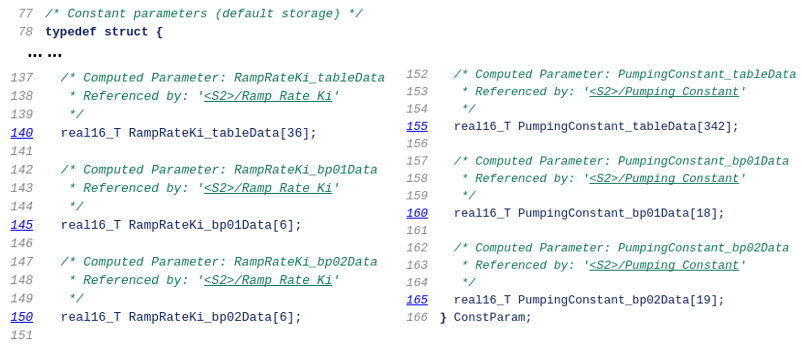```rmpath(expath); close_system('sldemo_fuelsys',0); ```

## Support평가판 신청